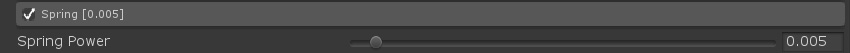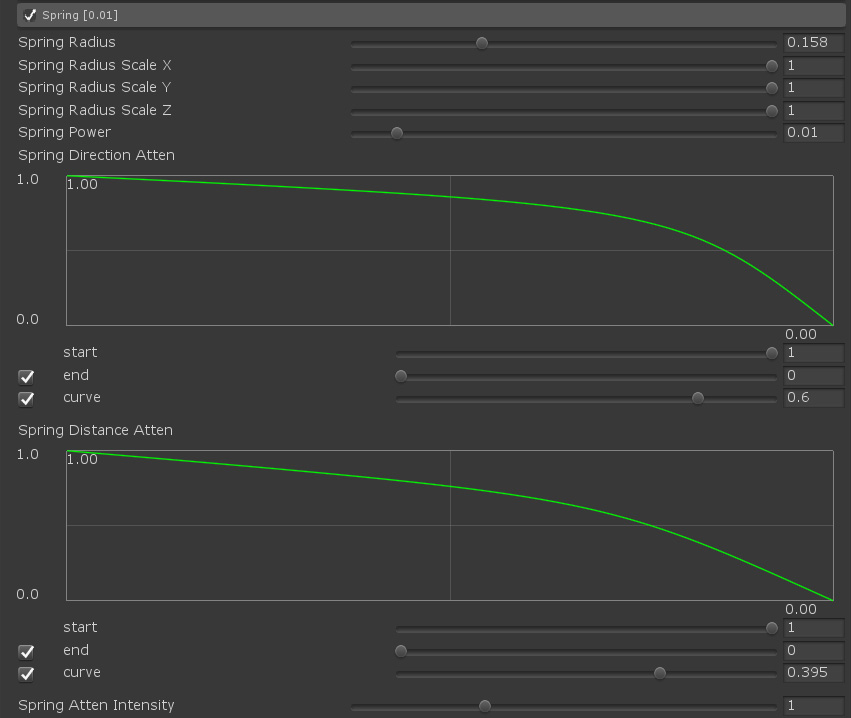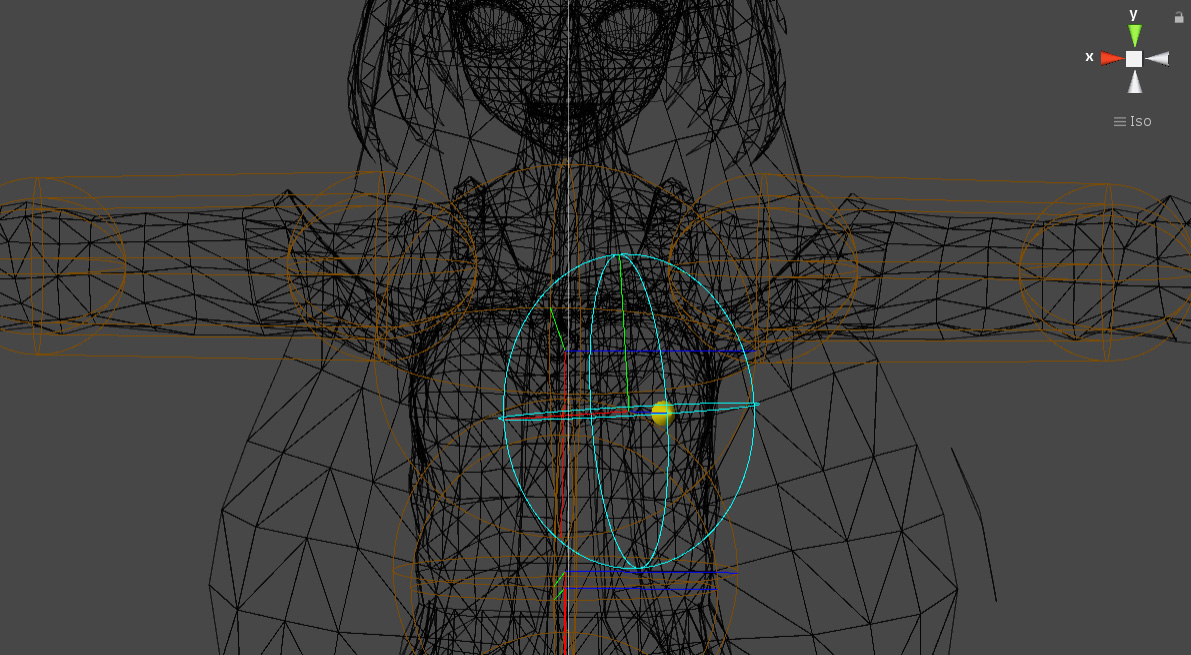# Spring

Spring parameters differ greatly between Bone Spring and Mesh Spring.

###### Bone SpringThe settings in BoneSpring are simple.

 Name Description Spring Power Spring power

###### Mesh SpringMesh Spring adds a spherical range setting that is affected by the spring.
A spherical area can be made elliptical by setting the radius and the scale of the XYZ axes.
In the following example, the range where the chest shakes is set with an ellipse.Each parameter is as follows:

 Name Description Spring Radius Radius of influence Spring Radius Scale X X scale of the range sphere Spring Radius Scale Y Y scale of the range sphere Spring Radius Scale Z Z scale of the range sphere Spring Power Spring power Spring Direction Atten Spring force damping rate depending on the angle from the front of the range sphere.This is a graph with the front direction on the left and the back direction on the right. Spring Distance Atten Spring force decay rate depending on distance from the center of the range sphere.A graph with the center of the range sphere on the left and the outer edge of the range sphere on the right. Spring Atten Intensity For overall adjustment of damping rate